Dynamics of Two Charged Particles in a Creeping Flow | OMICS International
Journal of Physical Mathematics

# Dynamics of Two Charged Particles in a Creeping Flow

Hassan HK and Stepanyants YA*

University of Southern Queensland, Toowoomba, Australia

*Corresponding Author:
Stepanyants YA
University of Southern Queensland
Toowoomba, Australia
E-mail: [email protected]

Received Date: August 06, 2015; Accepted Date: September 04, 2015; Published Date: September 11, 2015

Citation: Hassan HK, Stepanyants YA (2015) Dynamics of Two Charged Particles in a Creeping Flow. J Phys Math 6:145. doi:10.4172/2090-0902.1000145

Copyright: © 2015 Hassan HK, et al. This is an open-access article distributed under the terms of the Creative Commons Attribution License, which permits unrestricted use, distribution, and reproduction in any medium, provided the original author and source are credited.

Visit for more related articles at Journal of Physical Mathematics

#### Abstract

We study the interaction of two charged solid particles in a viscous fluid. It is assumed that the particles move either side-by-side or one after another along the same vertical line under the influence of the buoyancy/gravity force, Coulomb electrostatic force or its modification, and viscous drag force. The drag force consists of two components: the quasi-stationary Stokes drag force and Boussinesq–Basset drag force resulting from the unsteady motion. Solutions of the governing equations are analysed analytically and numerically for the cases of perfect fluid and viscous fluid; the comparison of these two cases is presented.

#### Keywords

Micro-particle; Viscous fluid; Charged particle; Stokes drag force; Boussinesq–Basset drag force creeping flow

AMS subject classification: 76D07, 70F05, 45A05, 45-04, 70-04

#### Introduction

Despite the fact that the study of particle dynamics in a viscous fluid has a long history, many important problems still remain unsolved . This pertains not only to the collective behaviour of big particle ensembles, but even to interactions of two particles in a complex environment of shear flows or in the presence of solid obstacles, walls or free surfaces. Much research efforts have been undertaken over the years to elucidate the particle dynamics in various situations. Importance of such investigations is backed by many practical applications of mixtures of fluids and suspensions; examples being particle motions in the cooling systems of nuclear reactors and particle-liquid mixtures used for pharmaceutical purposes. Other important examples are transport of particles (dusts and aerozoles) in the atmosphere and oceans (dynamics of suspensions, sand, biological products, etc.).

One of the intriguing problems that has not been solved so far is the dynamics of two inter- acting charged particles in viscous fluid. In recent years a great interest is observed to micro- and nano-particles due to their potential applications in modern biotechnologies and other micro-fluid technologies. Quantitative descriptions of such systems represent a certain challenge not only from the practical, but also from the academic point of view.

In this paper we consider elementary acts of interaction of two charged particles moving in a viscous fluid. We consider the interactions of non-conducting charged particles, when the electric charges are uniformly distributed within the spherical particles, and conducting particles, when the charges can freely move within the particles enhancing and reducing the Coulomb forces . The equations of motion are studied analytically where possible and numerically. The particle dynamics is considered in the creeping flow approximation, that is under the assumption that the Reynolds number is very small,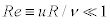, where u is particle velocity relative to the fluid, R is particle radius, and ν is fluid kinematic viscosity. For simplicity, we assume that particles are solid and have a spherical shape. We study two configurations of particles: i) when they move side-by-side perpendicular to the line connecting their centers and ii) when they move vertically one after another along the same line.

#### Equations of Motion and Problem Formulation

A motion of an individual uncharged particle in a viscous fluid at small Reynolds numbers in the creeping regime has been studied . It has been demonstrated that in the case of a transient flow the influence of Boussinesq–Basset drag (BBD) force [4-6] is very important. It provides different character of particle motions in comparison with the well-known Stokes drag (SD) force [7,8]. In the present paper we consider a motion of two electrically charged particles in different setups taking into account gravity/buoyancy force, electrostatic force and viscous drag forces. The effect of viscosity is taken into consideration through the SD force and BBD force which depends on the motion prehistory [4-6,8]. We also take into account a reciprocal influence of particles on the drag force which depends on the particle configuration . To our best knowledge the combined effect of all these factors were not studied thus far.

As a first step we consider two identical metallic particles with electric charges of the same absolute value (they can be either of like or unlike charges). As has been shown , the electrostatic force acting on metallic particles deviates from the classical Coulomb law: at small distances the force is not inversely proportional to the square of the distance between the particle centers. This deviation is important at relatively small distances between the particles while at large distances the electrostatic force asymptotically approaches the classical Coulomb law. An exact expression for the electrostatic force Fs as derived  and its asymptotic Coulomb approximation Fc are as follows,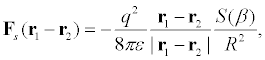(2.1)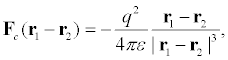(2.2)

where R is the particle radius, q is the value of the electric charge, ε is the permittivity of the medium,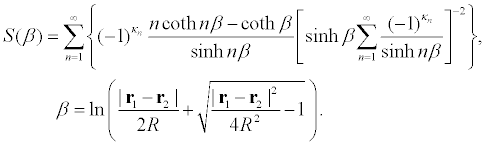κn=n + 1 for like charged particles and κn=0 for unlike charged particles. The physical reason of deviation of electrostatic force from the classical Coulomb formula is explained by redistribution of electric charges within the conducting spheres as illustrated by Figure 1. When electric charges can freely move within conducting spheres they either attract to each other if spheres unlikely charged or repeal from each other when the spheres likely charged.

Figure 1: Electric charge redistribution within two conducting unlikely charged spheres (a) and two unlikely charged spheres with uniformly distributed carges (b).

Figure 2 shows the dependences of the attractive and repulsive electrostatic forces normalized by q2/(16πεR2) as described by Equation (2.1) and the corresponding Coulomb forces under the same normalization as described by Equation (2.2). As one can see from this figure, corrections to the Coulomb forces become notable only when the distance between the particle centres is less than 4R. The modified attractive force infinitely increases when particles approach each other (see line1 in the Figure 2).

Figure 2: (color online) Normalized electrostatic force versus normalized distance, ξ=x/R, between two like (line 1) and unlike (line 2) charged particles as per Eq. (2.1). Dashed lines show Coulomb approximations as per Eq. (2.2).

When a solid particle moves in a viscous fluid, it experiences an influence of a drag force [7,8]. In the presence of another particle the drag force modifies and depends on many factors, including particle shapes, distance between them, their reciprocal orientation and velocities . The correction to the quasi-stationary SD force acting on particle in the presence of another particle can be taken into account through the effective viscosityν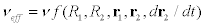, where ν is the usual coefficient of dynamic viscosity, and f is a rather complicated function of its arguments. This function gradually reduces to unity when the distance between the particles becomes much greater than their radii.

To the best of our knowledge, modifications of the transient BBD force exerting on a particle in the presence of another particle have not been studied yet. With this in mind, we can consider two notional possibilities: (a) there is no correction to the BBD force due to the presence of another particle, so that the BBD force remains the same as for a single particle; and (b) the correction to the BBD force is described by the same effective viscosity as the SD force. In what follows, both these possibilities will be explored and results will be compared.

Consider further two spherical particles moving in a viscous fluid in the creeping flow regime. The equation of motion of one spherical particle with the added mass effect taken into account is [7,8]: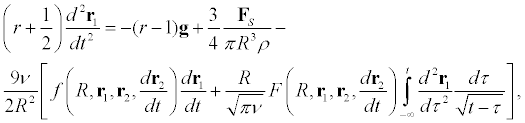(2.3)

where g is the acceleration due to gravity, ρ is the particle density, r is the particle-to-fluid density ratio. The added mass effect is taken into account through the coefficient 1/2 in the brackets in the left-hand side of the equation, the first term in the right-hand-side describes the gravity/buoyancy force, second term describes the electrostatic force and the third term describes the total drag force including the SD force (the first term in the square brackets) and the BBD force (the second, integral, term in the square brackets). Function F ≡ 1 if the correction to the BBD force is ignored, or F ≡ f if the correction to the BBD force is the same as for the SD force.

The same equation with the indices interchanged holds for the second particle. Subtracting and summing the equations for the individual particles, we obtain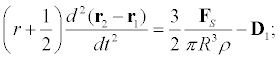(2.4)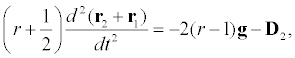(2.5)

where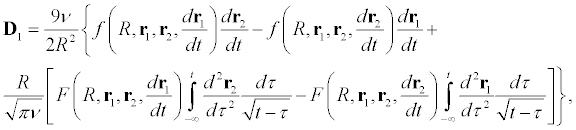(2.6)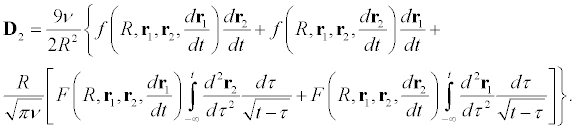(2.7)

Below we consider two particular cases of particle configuration when they move (i) side-by- side as sketched in Figure 3a and (ii) one after another as shown in Figure 3b

Figure 3: Two particles moving side-by-side in the direction normal to the line connecting their centers (a) and one after another along the line connecting their centers (b).

Two particles moving side-by-side

Considering the case of two particle moving side-by-side as shown in Figure 3a and assuming that the center of masses of the system does not move in the horizontal direction, we write down the scalar projections of Equations (2.6) and (2.7) onto the horizontal, x, and vertical, z, axes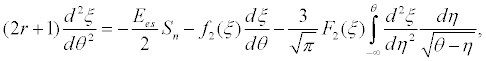(3.1)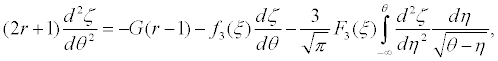(3.2)

Where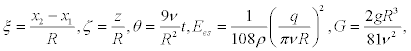and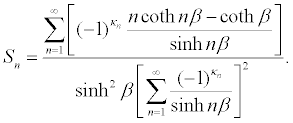Functions f1(ξ), f2(ξ), f3(ξ), as well as F1(ξ), F2(ξ), and F3(ξ) account for the reciprocal influence of particles on the drag forces exerted on them . Functions f1, f2 and f3 can be presented in terms of ξ1=1/(2ξ) as follows.

• In the case when two particles move with equal speeds in the same direction along the line connecting their centers: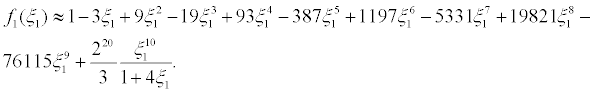(3.3)

• In the case when two particles move with equal speeds on absolute value in the opposite directions along the line connecting their centers: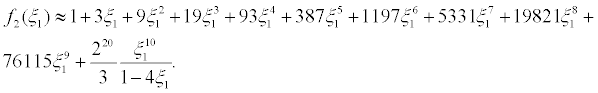(3.4)

• In the case when two particles move with equal speed in the same direction side-by-side in the direction perpendicular to the line connecting their centers and can freely rotate: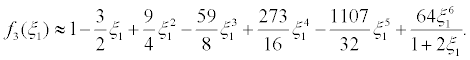(3.5)

As have been mentioned above, expressions for functions F1,2,3(ξ) are not known thus far, therefore we will consider two cases, when F1,2,3(ξ) ≡ 1 and when F1,2,3(ξ) ≡ f1,2,3(ξ). Functions f1,2,3(ξ) asymptotically approach unity when ξ → ∞, and the corresponding drag force reduces to the drag forces exerted on an isolated particles. However, when ξ → 2, which corresponds to the minimum distance between the particle centers (when the particles touch each other), functions f1(ξ) and f3(ξ) go to the finite limits: f1(2)=0.647 and f3(2)=0.694, whereas function f2(ξ) grows infinitely, f2(ξ) → ∞ as ξ → 2+. Physically unacceptable behaviour of the drag forces in this case is the consequence of the approximate character of the formula. Nevertheless, as noted , “Hocking states that good agreement on collision efficiencies is obtained with his results and experimental data, so it is apparent that under some conditions the approximate treatment is satisfactory”. The dependences f1,2,3(ξ) are shown in Figure 4.

Figure 4: (color online) Functions f1,2,3(ξ) versus ξ. Horizontal dashed line shows the asymptotic value for both functions when the particles are far away from each other. Vertical dashed line shows the minimal distance between the particles, ξmin=2.

Note that in the Coulomb approximation, when the distance between the particles is much longer than their radii or when the charges of spherical particles are localized in their centers, the first term in Equation. (2.6) takes the simple form Ees2. We will study the particle interaction in both cases, with exact formula for the electrostatic force, Fs (2.1), and with the Coulomb approximation for the electrostatic force Fc (2.2).

Equation (3.1) is independent of (3.2) and can be investigated separately. Once its solution is found and ξ(θ) is determined, Equation (3.2) can be solved then (notice that (3.2) contains ξ(θ) via functions f3(ξ) and F3(ξ)).

For computations we used the following values of parameters: water density ρ=103 kg/m3, water kinematic viscosity ν=6.05 • 10-7 m2/s, particle radius R=5 • 10-5 m=50 μ, charge values are equal to q=1.6 • 10-13C, water permittivity ε=6.954 • 10-10 F/m, particle-to-water density ratio r=2.7 (this corresponds to the aluminium mote). Based on these parameters, the dimensionless parameters are Ees=3.784 • 10−2 and G=8.272 • 10−2.

Particle dynamics in an in viscid fluid

Assuming that two particles with equal charges on absolute value are initially in the rest, let us study first the reference case, when the fluid is perfect and viscosity is absent (formally we put f1,2,3(ξ)=F1,2,3(ξ) ≡ 0). Then, Equation. (3.1) in the Coulomb approximation can be solved analytically; solutions for the like and unlike charged particles can be presented in the implicit forms,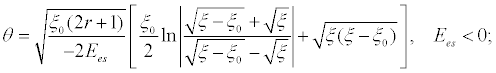(3.6)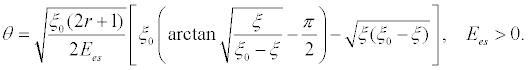(3.7)

The former solution corresponds to the repulsive case when ξ > ξ0, whereas the latter corresponds to the attractive case when ξ < ξ0.

Solution of Equation. (3.2) without viscosity is trivial – it is simply the motion from the rest with the constant acceleration,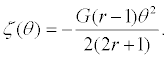Eliminating θ from the expressions ξ(θ) and ζ(θ), we obtain particle trajectories in both cases of particle repulsion or attraction: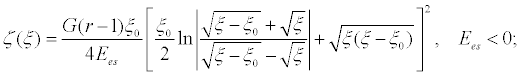(3.8)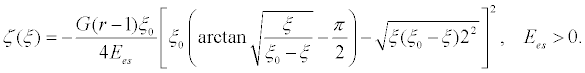(3.9)

Solution of Equation (3.1) beyond the Coulomb approximation can be readily obtained numerically. The equation was integrated by the fourth-order Runge–Kutta method with the fixed integration step using Mathcad-14 software. The infinite sums in Equation. (3.1) were replaced by finite series containing N=200 terms. The results for attractive and repulsive particles are shown in Figure 5 by solid lines 1 and 1′ (trajectories for repulsive particles are labelled by dashed numbers and go to the right, whereas trajectories for attractive particles to the left). In that figure we also show the analytical results obtained in the Coulomb approximation as per Equations. (3.8) and (3.9); they are shown by dashed lines 2 and 2′. Lines 3, 3′ and 4, 4′ pertain to the case of viscous fluid when both viscosity coefficients, for SDF and BBDF, are equally modified by functions f2(ξ) (3.4) for the horizontal motion and f3(ξ) (3.5) for the vertical motion (the detailed discussion of the viscosity effect will be presented in the next subsection). Lines 3 and 3′ pertain to the case of exact electrostatic force, and lines 4 and 4′ pertain to the Coulomb approximation. In all cases shown in the figure the particles started to move from the rest when the distance between them was 4 in the dimensionless units. Attractive particle collision occurs when the distance between them ξ=2.

Figure 5: (color online) Particle trajectories: vertical position of particles, ζ, against the distance between them, ξ, in the dimensionless variables. For explanation see the text.

As one can see from this figure, the particles collide in a finite time, when they are attracted by each other due to electrostatic force. The collision occurs sooner when the exact electrostatic force is taken into consideration compared to the case of Coulomb approximation. Accordingly, the vertical distance travelled by the particles before they collide is less for the former case compared to the latter case (cf. the trajectories 1 and 2 and 3 and 4 in Figure 5).

The situation is opposite when the particles repulse each other in the perfect fluid: in the case of exact electrostatic force the horizontal motion is slower than in the case of Coulomb approximation. Therefore, trajectory 1′ in the former case lies below the trajectory 2′ in the latter case. The repulsive particles move away from each other to infinity. The observed motion is the direct result of the difference between the exact electrostatic forces in comparison with the Coulomb force. The exact force is larger than the Coulomb force for the attractive particles, but smaller than the Coulomb force for the repulsive particles (Figure 2). When the distance between particles becomes large, the exact electrostatic force quickly reduces to the Coulomb force (Figure 2). However, if particles start moving at a relatively small distance between them, the time lag of trajectory 2′ relative to trajectory 1′ still occurs.

In the viscous fluid repulsive particles move faster in horizontal direction when the Coulomb approximation is used. Therefore, trajectory 4′ lies below the trajectory 3′ which corresponds to exact electrostatic force. This is, apparently, a consequence of a complicated character of modified BB drag with the variable viscosity coefficient νeff. We will revert to this issue in the next subsection.

Figure 6 illustrates the variation of relative particle velocity in horizontal direction with the distance between them. When particles attract each other their relative speed at the moment of collision (at ξ=2) is higher when the exact electrostatic force is considered then in the case of Coulomb approximation (cf. lines 1 and 2 in Figure 6). When particles repeal from each other, their relative speed varies with distance almost equally both in the case of exact electrostatic force and in the Coulomb approximation; therefore lines 1′ and 2′ are practically indistinguishable.

Figure 6: (color online) Relative horizontal velocity of particles against the distance between them in the dimensionless variables. Curve numbering corresponds to Figure 5. The vertical scale for the viscous case represented by lines 3, 4, 3′, and 4′ is shown on the right.

In viscous fluid horizontal speed of particles always greater when the exact electrostatic force is used in comparison with the Coulomb force (cf. lines 3 and 4, as well as lines 3′ and 4′. Notice that the vertical scale for the viscous case represented by lines 3, 4, 3′, and 4′ (shown on the right) is 20 times greater than in the inviscid case (shown on the left) represented by lines 1, 2, 1′, and 2′.

Particle dynamics in viscous fluid

The description of particle dynamics becomes much more complicated when the viscosity is taken into account. The simplest case is the motion of uncharged particles with Ees=0. Consider first the case when the initial distance between the particles is so large that functions f1,2,3(ξ) and F1,2,3(ξ) can be replaced by unities. In such case the set of equations (3.1)–(3.2) can be solved analytically , however, the solution is quite cumbersome. Here we only present the universal asymptotic form of solution for large θ assuming that particles commence motion with zero vertical velocities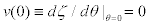, but with non-zero relative horizontal velocity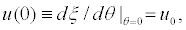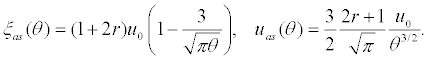(3.10)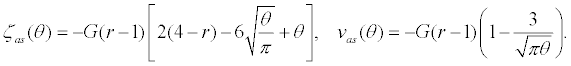(3.11)

If the distance between the particles is not large enough, then functions f1,2,3(ξ) and F1,2,3(ξ) cannot be replaced by unities. None of Equations (3.1) and (3.2) is integrable in this case. Equations (3.1) and (3.2) were integrated numerically by means of Fortran code using the fourth-order Runge–Kutta scheme and the standard RKGS subroutine in Gill’s modification. The infinite sums in Equation (3.1) were replaced by finite series with N=400 terms. The numerical code has been tested against the exact analytical solutions  and demonstrated quite reliable results. Examples of numerical solutions of Equations (3.1)– (3.2) with different models of viscosity are presented in Figure 7.

Figure 7: (color online) Particle trajectories in viscous fluid with different models of viscosity. For explanations see the text.

Lines 3, 3′ and 4, 4′ are the same as in Figure 5, i.e. line 3 represents the trajectory of attractive particles when exact electrostatic force is considered and viscosity coefficients are modified in accordance with Equations (3.4) for the horizontal motion and (3.5) for the vertical motion. Line 4 represents the trajectory when the Coulomb approximation is used for electrostatic force. Lines 3′ and 4′ represent the trajectories for the repulsive particles with exact electrostatic force and in the Coulomb approximation correspondingly. As one can see, in the repulsive case trajectories 3′ and 4′ are fairly close to each other, whereas in the attractive case the difference between them is quite noticeable at small distances between the particles. In what follows we consider only exact electrostatic force.

Line 1 (1′) represents particle trajectory in the attractive (repulsive) case when only SD force is taken into consideration with the constant viscosity, i.e. when the influence of another particle is ignored, as well as influence of the BBD force.

Line 5 (5′) represents particle trajectory in the attractive (repulsive) case when only SD force is taken into consideration with the modified viscosity as per Equations (3.4) and (3.5) when the influence of the BBD force is ignored.

Line 2 (2′) represents particle trajectory in the attractive (repulsive) case when the SD force is taken into consideration with the modified viscosity as per Equations (3.4) and (3.5), whereas the viscosity coefficient for the BBD force is assumed constant F2,3=1.

As one can see from these graphics, the model with only SD force with constant viscosity pro-videos the results which significantly differ from the results of other models with variable viscosity and BBD force. In the meantime, lines 5 and 5′ are very close to lines 3 and 3′ correspondingly.

This indicates that the BBD force does not play a significant role in comparison with SDF in such motions and hence can be neglected. Figure 8 illustrates the variation of relative particle velocity in the horizontal direction with a distance between them. Line labels in this figure correspond to labels of trajectories in Figure 7. Only the vertical scale of line 5 (but not line 5′!) is 10 times compressed in comparison to all other lines.

Figure 8: (color online) Relative horizontal velocity of particles against the distance between them in the dimension less variables. Curve numbering corresponds to Figure 7. The vertical scale for lines 1 and 1′ are shown on the right. Labels for lines 2′ and 3′ are not shown; these two lines are very close to each other and are disposed between the lines 4′ and 5′.

Observe that qualitatively the difference between the exact and Coulomb cases is similar to that shown in Figure 5. Namely, for the attractive particles the horizontal motion is faster when the exact electrostatic force is considered, while for the repulsive particles the horizontal motion is a bit faster when the Coulomb electrostatic force is used.

It is interesting that at the initial stage of motion the relative horizontal speed increases very rapidly, then the speed continue increasing, but with the moderate rate, and then after reaching a maximum value it decreases due to strong influence of drag force correction f2(ξ) caused by the close presence of the second particle. If such correction is ignored, then the relative horizontal speed monotonically increases until particle collision (cf. line 5 with other lines 1-4 in Figure 7).

As have been noted, in the attraction case the relative horizonatl speed is high for the case of exact electrostatic force in comparison to Coulomb approximation. It is also interesting to note that both the dragcorrection factor f2(ξ) and electrostatic force infinitely increase when the attractive particles approach each other (when ξ → 2). However, the influence of variable viscous term prevails over electrostatic force resulting in the speed deceleration at moment of collision.

#### Two Particles Moving One After Another

Consider now the case when two particles move one after another as shown in Figure 3b). Equations of motion in the scalar dimensionless form follow again from Equations (2.3):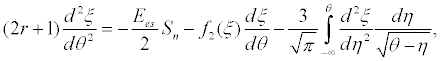(4.1)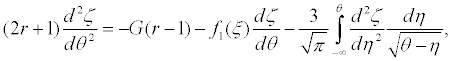(4.2)

now ζ=(z1 + z2)/2R is the dimensionless coordinate of the mass center, and other dimensionless quantities are defined after Equation (3.2). Function f1(ξ) is defined in Equations (3.3). Equation (4.1) describes time variation of the relative distance between the particles; it is exactly the same as Equation. (3.1, whereas Equation. (4.2) slightly differs from Equation. (3.2) due to replacement of function f3(ξ) by function f1(ξ). The difference between these two functions is not too big, as one can see from Figure 4, therefore solution of the set (4.1, 4.2) does not differ too much from the solution of the set (3.1, 3.2).

In Figure 9 we present a comparison of two trajectories when the exact electrostatic force was used with the modified viscosity coefficient of SDF only, whereas BBDF was taken with the constant coefficient. Lines 5 and 5′ pertain to the case when particles move side-by-side, and lines 6 and 6′ pertain to the case when particles move vertically one after another. In the later case the drag force for the motion of mass center is less than in the former case (f1(ξ) < f3(ξ)), therefore the traversed path by mass center before the particle collision in the later case (z ≈ −166.5) is a bit greater than in the former case (z ≈ −148.5).

Figure 9: (color online) Particle trajectories moving side-by-side (lines 5 and 5′) and vertically one after another (lines 6 and 6′) in a viscous fluid. For the detailed explanations.

Due to Equations (3.1) and (4.1) are the same, the relative particle velocities are equal in the corresponding cases of side-by-side and vertical motions (see lines 5, 5′ and 6, 6′ in Figure 10). But the vertical motion of mass centers in these two cases is slightly different due to the difference in Equations (3.2) and (4.2) (see lines 7, 7′ and 8, 8′ in Figure 10).

Figure 10: (color online) Relative velocity and the velocity of mass center of two particles against the distance between them in the dimensionless variables. Lines 5, 6 and 5′, 6′ pertain to the relative velocity of attractive and repulsive particles. Lines 7, and 7′ pertain to the side-by-side vertical motion of two particles, and lines 8, and 8′ pertain to the vertical motion of two particles one after another. For the detailed explanations see the text. The vertical scale for lines 7, 8, 7′, and 8′ is shown on the right.

#### Discussion and Conclusion

We have considered the dynamics of two unlike and like charged particles in viscous fluid in the creeping flow approximation. Relative particle dynamics have been studied under different models of electrostatic force acting between the particles: the force between two conducting spheres as derived by Saranin  and Coulomb’s force between point-like particles. Two types of viscous drag forces were taken into consideration: the quasi-stationary Stokes drag force and the transient Boussinesq–Basset drag force. Different models of viscous drag forces were analysed, when the viscosity coefficient is constant, like in the case of a single particle, and when it is modified due to the presence of a second particle. Comparison of particle trajectories under the influence of all these forces were studied and compared with the case of inviscid fluid.

Using the typical value of parameters (see the paragraph before the subsection 3.1.), we obtain that two aluminium micro-particles of a radii 50 μ approaching each other from the distance 200 μ traverse 7.5 mm in the vertical direction before the collision. The maximal relative velocity between the particles is ∼ 0.44 mm/s, and their vertical velocity attains ∼ 2.28 cm/s.

Results obtained can be useful for the development of control methods of micro-and nano-particle dynamics in viscous fluids in application to technological processes and medicine .

#### References

Select your language of interest to view the total content in your interested language

### Article Usage

• Total views: 13215
• [From(publication date):
November-2015 - Feb 23, 2020]
• Breakdown by view type
• HTML page views : 9275Can't read the image? click here to refresh